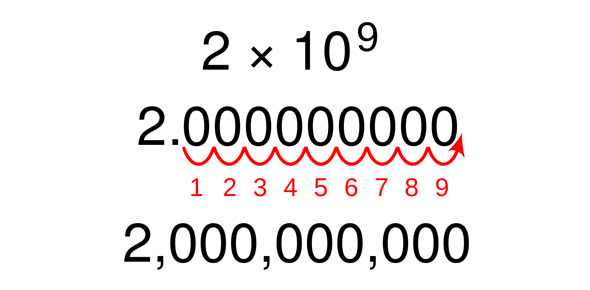# Scientific Notation Quiz Questions

6 Questions | Total Attempts: 260SettingsIf you get a good grade on this quiz you are prepared to take the test on this section.

• 1.
1*1022=
• A.

100000000000000000000

• B.

1000000000000000000000 10000000000000000000000.0

• C.

1.0000000000000000000000

• D.

1.1

• 2.
18,000,000=
• A.

1.8*10^7

• B.

18*10^0

• C.

180*10^7

• D.

1800*10^8

• 3.
Mark t if statement is true and f if its false 17,000,000=170*106
• A.

True

• B.

False

• 4.
12*106=12,000,000
• A.

True

• B.

False

• 5.
1*1022
• A.

Standard

• B.

Scientific

• C.

Non

• 6.
12*1010
• A.

Standard

• B.

Scientific

• C.

Non

Related TopicsBack to top